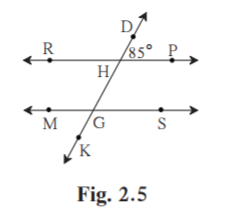No products in the cart.

Ask questions which are clear, concise and easy to understand.

Kulbeer Bhandari 2 years, 2 months ago

Part

Gaurav Seth 2 years, 5 months ago

1. In figure 2.5, line RP || line MS and line DK is their transversal. DHP = 85° Find the measures of following angles.

(i) RHD (ii) PHG (iii) HGS (iv) MGKSolution:

Given RPMS.

DK is the transversal.

(i) DHP = 85˚

RHD = 180-85 = 95˚ [Linear pair]

(ii) PHG = RHD = 95˚ [RHD and PHG are vertically opposite angles]

(iii)HGS = 180-95 = 85˚ [PHG and HGS are corresponding interior angles]

(iv)MGK = HGS = 85˚ [Vertically opposite angles]

Abujar Gour 2 years, 5 months ago

Bd

Yogita Ingle 3 years ago

P = 10800
r =25/2 (annually)
number of years n = 3 yrs
A = p{1+r/100}n
= 10800{1+25/200}3
=rs 15377.34 (approx)
CI = A - P
= rs(15377.34 - 10800)
= rs 4577.34## myCBSEguide

Trusted by 1 Crore+ Students## Test Generator

Create papers online. It's FREE.## CUET Mock Tests

75,000+ questions to practice only on myCBSEguide app